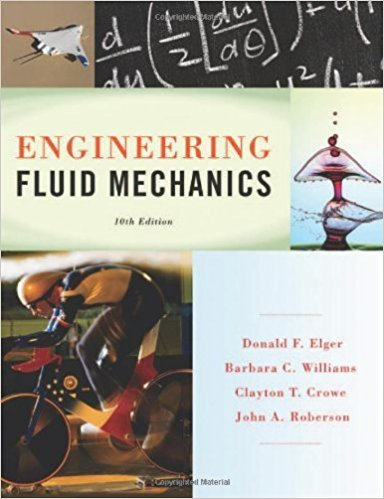×
×

# Apply the modeling building process to the Balloon Payload problem described here. aISBN: 9781118164297 288

## Solution for problem 16.2 Chapter 16

Engineering Fluid Mechanics | 10th Edition

• Textbook Solutions
• 2901 Step-by-step solutions solved by professors and subject experts
• Get 24/7 help from StudySoup virtual teaching assistantsEngineering Fluid Mechanics | 10th Edition

4 5 1 272 Reviews
27
3
Problem 16.2

Apply the modeling building process to the Balloon Payload problem described here. a. What are the relevant variables? b. How are the variables related? What are the relevant equations? How can you apply these equations to develop a single algebraic equation to solve for your goal? c. What is a simple and low-cost way to test your math model using experimental data?

Step-by-Step Solution:
Step 1 of 3

Recall: The Electric Field §A charge creates an electric field around itself and the other charge feels that field. + + Test charge q 3 Review - Point Charge The electric field created by a point charge, as a function...

Step 2 of 3

Step 3 of 3

##### ISBN: 9781118164297

Unlock Textbook Solution